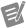Sign (G Dataflow)

Returns the sign of the input value.Note

For nonzero complex numbers, this node returns a complex value with the same phase as the input and with a magnitude of 1.number

An input to this operation.

This input supports waveforms and any data type that contains only numbers, such as scalar numbers, arrays or clusters of numbers, and arrays of clusters of numbers.sign

The sign of the input value. sign returns the following values.

 -1 number is less than 0. 0 number is equal to 0. 1 number is greater than 0.
When number is in the form of a + b i, that is, when number is complex, the following equation defines sign:
$\frac{a+bi}{|a+bi|}$

Where This Node Can Run:

Desktop OS: Windows

FPGA: DAQExpress does not support FPGA devices